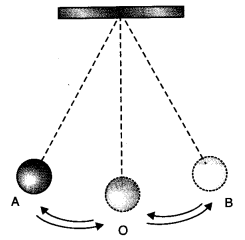# CBSEtips.in

## Thursday, 25 February 2021

### CBSE Class 7 Science - MCQ and Online Tests - Unit 13 - Motion and Time

#### CBSE Class 7 Science – MCQ and Online Tests – Unit 13 – Motion and Time

Every year CBSE students attend Annual Assessment exams for 6,7,8,9,11th standards. These exams are very competitive to all the students. So our website provides online tests for all the 6,7,8,9,11th standards’ subjects. These tests are also very effective and useful for those who preparing for any competitive exams like Olympiad etc. It can boost their preparation level and confidence level by attempting these chapter wise online tests.

These online tests are based on latest CBSE syllabus. While attempting these, our students can identify their weak lessons and continuously practice those lessons for attaining high marks. It also helps to revise the NCERT textbooks thoroughly

#### CBSE Class 7 Science – MCQ and Online Tests – Unit 13 – Motion and Time

Choose the correct answer:

Question 1.
Time period of a simple pendulum depends upon its
(a) weight of bob
(b) length
(c) both (a) and (b)
(d) None of these

Question 2.
The correct symbol to represent the speed of an object is:
(a) 5 m/s
(b) 5 mp
(c) 5 m/s-1
(d) 5 s/m

Answer: (d) 5 s/m

Question 3.
If we denote speed by S, distance by D and time by T, the relationship between these quantities is
(a) S = D T
(b) T = $$\frac{S}{D}$$
(c) S = $$\frac{1}{T}$$ × D
(d) S = $$\frac{T}{D}$$

Answer: (c) S = $$\frac{1}{T}$$ × D

Question 4.
Observe the figure given below:The time period of a simple pendulum is the time taken by it to travel from
(a) A to B and back to A
(b) O to A, A to B and B to A
(c) B to A, A to B and B to O
(d) A to B

Answer: (a) A to B and back to A

Question 5.
Nearly all the clocks make use of
(a) straight line motion
(b) periodic motion
(c) random motion
(d) circular motion

Answer: (b) periodic motion

Question 6.
A simple pendulum takes 42 sec. to complete 20 oscillations. What is its time period?
(a) 2.1 s
(b) 4.2 s
(c) 21 s
(d) 8.40 s

Answer: (a) 2.1 s

Question 7.
Boojho walks to his school which is at a distance of 3 km from his home in 30 minutes. On reaching he finds that the school is closed and comes back by a bicycle with his friend and reaches home in 20 minutes. His average speed in km/h is
(a) 8.3
(b) 7.2
(c) 5
(d) 3.6

Question 8.
A bus travels 54 km in 90 minutes. The speed of the bus is
(a) 0.6 m/s
(b) 10 m/s
(c) 5.4 m/s
(d) 3.6 m/s

Answer: (b) 10 m/s

Question 9.
On which axis is dependent variable represented?
(a) x-axis
(b) y-axis
(c) On any axis
(d) Depends on the data

Question 10.
Which of the following cannot be used for measurement of time?
(a) A leaking tap
(b) Simple pendulum
(c) Shadow of an object during the day
(d) Blinking of eyes